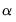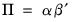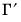New Features in EViews 13 : Command Language

Command Language
Updated Command List
Commands
dbopen open a database. (updated)
pageload load one or more pages into a workfile from a workfile or a foreign data source. (updated)
pagesave save page into a workfile or a foreign data source. (updated)
stom Converts series, alpha, or group to vector, svector, or matrix after removing observations with missing values. (updated)
stomna Converts series, alpha, or group to vector, svector, or matrix without removing observations with missing values. (updated)
wfdir change the workfile view to a simple object directory listing. (new)
wffilter change the workfile object filter for the current workfile window. (new)
wfopen open workfile or foreign source data as a workfile. (updated)
wfsave save workfile to disk as a workfile or a foreign data source. (updated)
xopen open a connection to an external application. (updated)
Updated Object List

Coef
Coef Procs
export save coef as Excel 2007 XLSX, CSV, tab-delimited ASCII text, RTF, HTML, Enhanced Metafile, PDF, TEX, or MD file on disk. (new)
import imports data from a foreign file into the coef object. (new)
Coef Values
@droprow(arg) Returns the coef with the rows defined by arg removed. arg may be an integer, vector of integers, string, or svector of strings. Integer values correspond to rows and string values correspond to previously defined row labels. (new)
@row(arg) Returns the rows defined by arg. arg may be an integer, vector of integers, string, or svector of strings. Integer values correspond to rows and string values correspond to previously defined row labels. (new)
Equations
Equation Methods
ardl least squares with autoregressive distributed lags. (updated)
did estimate a panel equation using the difference-in-difference estimator. (new)
ls equation using least squares or nonlinear least squares. (updated)
Equation Views
boundstest perform the Pesaran, Shin and Smith (2001) bounds test of long-run relationships from an ARDL estimated equation. (updated)
cointrel display information about the cointegrating relation specification and the coefficients in ARDL estimated equation. (new)
didcs compute Callaway-Sant’Anna decomposition for difference-in-difference estimation. (new)
didgbdecomp perform Goodman-Bacon decomposition for difference-in-difference estimation. (new)
didtrends show difference-in-difference trends summary in graphical or tabular form. (new)
dynmult compute dynamic multipliers for long-run regressors in ARDL equations. (new)
ecresults display the conditional error correction (CEC) and error correction (EC) regression results. (new)
similarity compute PMG Hausman test for similarity. (new)
symmtest compute symmetry test for nonlinear distributed lag variables in nonlinear ARDL models. (new)
Equation Procs
didmakeeq create an equation object with the underlying fixed-effects estimation of a difference-in-difference equation. (new)
fit static forecast. (updated)
forecast dynamic forecast. (updated)
Equation Values
@varselkept space delimited list of variables kept by model selection. (new)
@varselrejected space delimited list of the variables dropped by model selection. (new)
Geomap
Geomap Procs
setfillcolor define the fill (background) color used in geomap shapes using values in a series. (updated)
setjust set the display justification for multi-line area labels. (new)
setshapelabel set which attribute to use or create a custom label to use when labeling shapes. (new)
Geomap Values
@ids("attr", "val") space delimited string containing the ID numbers of all the areas which has the matching attribute value for the specified attribute name. (new)
Graph
Graph Procs
datalabel controls labeling of the observations/data in time plots. (new)
datelabel controls labeling of the bottom date/time axis in time plots. (updated)
setelem set individual line, symbol, bar and legend options for each series in the graph. (updated)
Group
Group Views
coint test for cointegration between series in a group. (updated)
Group Procs
setfillcolor set custom spreadsheet fill coloring for the group. (updated)
settextcolor set custom spreadsheet text coloring for the group. (updated)
Matrix
Matrix Procs
clearcollabels clear the column labels in a matrix object. (new)
clearrowlabels clear the row labels in a matrix object. (new)
export save matrix as Excel 2007 XLSX, CSV, tab-delimited ASCII text, RTF, HTML, Enhanced Metafile, PDF, TEX, or MD file on disk. (new)
import imports data from a foreign file into the matrix object. (updated)
resize resize the matrix object (new).
setcollabels set the column labels in a matrix object. (updated)
setrowlabels set the row labels in a matrix object. (updated)
Matrix Values
@col(arg) Returns the columns defined by arg. arg may be an integer, vector of integers, string, or svector of strings. Integer values correspond to rows and string values correspond to previously defined column labels. (updated)
@dropcol(arg) Returns the matrix with the columns defined by arg removed. arg may be an integer, vector of integers, string, or s svector of strings. Integer values correspond to rows and string values correspond to previously defined column labels. (new)
@droprow(arg) Returns the matrix with rows defined by arg removed. The arg may be integer, vectors of integers, strings, or svectors of strings. Integer values correspond to rows and string values correspond to previously defined row labels. (new)
@dropboth(arg1, arg2) Returns the matrix with the rows defined by arg1 and columns defined by arg2 removed. The args may be integers, vectors of integers, string, or svectors of strings. Integer values correspond to rows and string values correspond to previously defined row labels. (new)
@row(arg) Returns the rows defined by arg. arg may be an integer, vector of integers, string, or svector of strings. Integer values correspond to rows and string values correspond to previously defined row labels. (updated)
@sub(arg1, arg2) Returns the matrix with rows defined by arg1 and columns with defined by arg2. The args may be integers, vectors of integers, strings, or svectors of strings. Integer values correspond to rows and string values correspond to previously defined row labels. (updated)
Rowvector
Rowvector Procs
clearcollabels clear the column labels in a rowvector object. (new)
clearrowlabels clear the row labels in a rowvector object. (new)
export export vector as Excel 2007 XLSX, CSV, tab-delimited ASCII text, RTF, HTML, Enhanced Metafile, PDF, TEX, or MD file on disk. (new)
import imports data from a foreign file into the vector object. (updated)
resize resize the rowvector object. (new)
setcollabels set the column labels in a rowvector object. (new)
setrowlabels set the row labels in a rowvector object. (new)
Rowvector Values
@col(arg) Returns the columns defined by arg. arg may be an integer, vector of integers, string, or svector of strings. Integer values correspond to rows and string values correspond to previously defined column labels. (new)
@dropcol(arg) Returns the matrix with the columns defined by arg removed. arg may be an integer, vector of integers, string, or svector of strings. Integer values correspond to rows and string values correspond to previously defined column labels. (new)
@t Returns transpose. (new)
Series
Series Procs
autoarma forecast from a series using an ARIMA model with automatic determination of the specification. (updated)
dsa seasonally adjust daily data using the DSA method. (new)
ets perform Error-Trend-Season (ETS) estimation and exponential smoothing (here). (updated)
forcavg average forecasts of a series. (updated)
setfillcolor define the fill (background) color used in series spreadsheets. (updated)
settextcolor set custom spreadsheet text coloring for the series. (updated)
smooth exponential smoothing. (updated)
Svector
Svector Procs
clearcollabels clear the column labels in a svector object. (new)
clearrowlabels clear the row labels in a svector object. (new)
fill fill the svector with the specified values. (new)
resize resize the svector object. (new)
setcollabels set the column labels in a svector object. (new)
setrowlabels set the row labels in a svector object. (new)
Svector Values
@droprow(arg) Returns the svector with the rows defined by arg removed. arg may be an integer, vector of integers, string, or svector of strings. Integer values correspond to rows and string values correspond to previously defined row labels. (new)
@row(arg) Returns the rows defined by arg. arg may be an integer, vector of integers, string, or svector of strings. Integer values correspond to rows and string values correspond to previously defined row labels. (new)
Sym
Sym Procs
clearcollabels clear the column labels in a vector object. (new)
clearrowlabels clear the row labels in a vector object. (new)
export save sym matrix as Excel 2007 XLSX, CSV, tab-delimited ASCII text, RTF, HTML, Enhanced Metafile, PDF, TEX, or MD file on disk. (new)
import imports data from a foreign file into the sym object. (updated)
resize resize the sym object. (new)
setcollabels set the column labels in a sym object. (new)
setrowlabels set the row labels in a sym object. (new)
Sym Values
@col(arg) Returns the columns defined by arg. arg may be an integer, vector of integers, string, or svector of strings. Integer values correspond to rows and string values correspond to previously defined column labels. (new)
@dropcol(arg) Returns the matrix with the columns defined by arg removed. arg may be an integer, vector of integers, string, or svector of strings. Integer values correspond to rows and string values correspond to previously defined column labels. (new)
@droprow(arg) Returns the matrix with rows defined by arg1 and columns with rows defined by arg2 removed. The args may be integer, vectors of integers, strings, or svectors of strings. Integer values correspond to rows and string values correspond to previously defined row labels. (new)
@dropboth(arg) Returns the sym with the rows and columns defined by arg removed. arg may be an integer, vector of integers, string, or svector of strings. Integer values correspond to rows and string values correspond to previously defined row labels. (new)
@dropboth(arg1, arg2) Returns the matrix with the rows defined by arg1 and columns defined by arg2 removed. The args may be integers, vectors of integers, string, or svectors of strings. Integer values correspond to rows and string values correspond to previously defined row labels. (new)
@row(arg) Returns the rows defined by arg. arg may be an integer, vector of integers, string, or svector of strings. Integer values correspond to rows and string values correspond to previously defined row labels. (new)
@sub(arg) Returns the sym with rows defined by arg1 and columns with rows defined by arg2. The args may be integers, vectors of integers, strings, or svectors of strings. Integer values correspond to rows and string values correspond to previously defined row labels. (new)
@sub(arg1, arg2) Returns the matrix with rows defined by arg1 and columns with defined by arg2. The args may be integers, vectors of integers, strings, or svectors of strings. Integer values correspond to rows and string values correspond to previously defined row labels. (updated)
@t Returns transpose. (new)
Table
Table Procs
deletecells delete cells from a table. (updated)
deletecol remove columns from a table. (updated)
deleterow remove rows from a table. (updated)
fixcol fixes a set of columns to left of the spreadsheet view so that the leading columns are always in view. (new)
fixrow fixes a set of rows at the top of the spreadsheet view so that the leading rows are always in view. (new)
fixrowcol fixes a set of rows at the top and a set of columns to left of a spreadsheet view so that the leading rows and columns are always in view. (new)
insertcells insert cells into a table. (updated)
insertcol insert additional columns into a table. (updated)
insertrow insert additional rows into a table. (updated)
save save table as Excel 2007 XLSX, CSV, tab-delimited ASCII text, RTF, HTML, Enhanced Metafile, PDF, TEX, or MD file on disk. (updated)
setfillcolor set the fill (background) color of a set of table cells. (updated)
setfont set the font for the text in a set of table cells. (updated)
setformat set the display format of a set of table cells. (updated)
setheight set the row height in a set of table cells. (updated)
setjust set the justification for a set of table cells. (updated)
setlines set the line characteristics and borders for a set of table cells. (updated)
settextcolor set the text color in a set of table cells. (updated)
setwidth set the column width for a set of table cells. (updated)
String values
@find(“arg”) string containing the cell identifiers meeting the boolean argument. This argument can be a mathematical or string expression. For example:
string s = tab1.@find("[b1:c15]>0.3")
returns a list of cells between B1 and C15 greater than 0.3;
string s = tabl1.@find("[a1:e67]== ""1949q4""")
returns a list of cells between A1 and E67 matching the string ‘1949q4’ (string comparisons ignore case);
string s = tab1.@find("[@all]==0.5")
returns a list of cells in the table equal to 0.5;
string s = t.@find("@instr([@all],""in"")")
returns a list of cells in the table containing the substring ‘in’;
string s = t.@find("[b3:c5]<[d5]")
returns a list of cells between B3 and C5 less than cell D5;
string s = t.@find(“@left([@all],1)==""cp"")
VAR
Var Methods
btvcvar estimate a Bayesian time-varying coefficients VAR specification. (new)
ec estimate a vector error correction model. (updated)
Var View
coint Johansen cointegration test. (updated)
impulse impulse response functions. (updated)
vdecomp variance decomposition. (updated)
Var Procs
fit produce static forecasts from an estimated VAR. (updated)
forecast produce dynamic forecasts from an estimated VAR or VEC. (updated)
Var Values
@cointadj matrix containing cointegrating variable adjustment coefficients(where applicable).
@cointadjse matrix containing standard errors of cointegrating variable adjustment coefficients (where applicable).
@cointlr matrix containing long-run equilibrium coefficients(where applicable)
@cointlrse matrix containing standard errors of long-run equilibrium coefficients (where applicable)
@cointsr matrix containing short-run adjustment coefficients(where applicable).
@cointsrse matrix containing standard errors of short-run adjustment coefficients (where applicable).
Vector
Vector Procs
clearcollabels clear the column labels in a vector object. (new)
clearrowlabels clear the row labels in a vector object. (new)
export export vector as Excel 2007 XLSX, CSV, tab-delimited ASCII text, RTF, HTML, Enhanced Metafile, PDF, TEX, or MD file on disk. (new)
import imports data from a foreign file into the vector object. (updated)
resize resize the vector object. (new)
setcollabels set the column label for the vector object. (updated)
setrowlabels set the row labels for the vector object. (updated)
Vector Values
@droprow(arg) Returns the vector with the rows defined by arg removed. arg may be an integer, vector of integers, string, or svector of strings. Integer values correspond to rows and string values correspond to previously defined row labels. (new)
@row(arg) Returns the rows defined by arg. arg may be an integer, vector of integers, string, or svector of strings. Integer values correspond to rows and string values correspond to previously defined row labels. (updated)
@t Returns transpose. (new)
Updated Function List
String Functions
@sfill Returns a svector containing the elements specified by the arguments to the function. (new)
@str returns a string representing the given number. (updated)
@val returns the number that a string represents. (updated)
@wreplace replaces parts of a string based on patterns. (updated)
Matrix Functions
@grid Vector containing an equally spaced grid of elements. (new)
@hcat Horizontally concatenate matrix objects. (updated)
@range Returns a vector holding the sequential integers staring at l and ending at h. (new)
@vech Vectorize (stack columns of) lower triangle of matrix object. (updated)
@zeros Matrix or vector of zeros. (new)
Support Functions
@makevalidname string containing an uppercased valid EViews name based on the input. (updated)
@xtype returns the string describing the type of the active external application. (new)
Workfile Functions
@event event identifier for observation (see “Event Function (@event)”). (updated)
@holiday returns the proportion of an annual event covered by each observation (see “Holiday Functions”). (updated)
@holidayset return the proportion of an annual set of events covered by each observation (see “Holiday Functions”). (updated)
Cumulative Statistics Functions
@cumdn cumulative process of negative (below threshold) changes. (new)
@cumdp cumulative process of positive (above threshold) changes. (new)
@cumdz cumulative process of zero (at threshold) changes. (new)
@dcumdp difference of cumulative process of positive (above threshold) changes. (new)
@dcumdn difference of cumulative process of negative (below threshold) changes. (new)
@dcumdz difference of cumulative process of zero (at threshold) changes. (new)